﻿ 强红外热辐射背景下的成像仿真 Imaging Simulation under the Background of Strong Infrared Thermal Radiation

Modeling and Simulation
Vol. 08  No. 02 ( 2019 ), Article ID: 30475 , 13 pages
10.12677/MOS.2019.82009

Imaging Simulation under the Background of Strong Infrared Thermal Radiation

Yingnan Si, Ye Li, Dan Yue*

Changchun University of Science and Technology, Changchun Jilin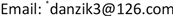Received: May 8th, 2019; accepted: May 21st, 2019; published: May 28th, 2019ABSTRACT

Due to the friction of the atmosphere and the convection heat transfer effect of the surrounding flow field, the temperature of the fairing increases, and the aerodynamic thermal radiation effect generated by it is received by the infrared sensor, forming radiation interference, which increases the background brightness and reduces the system’s ability to detect and track the target. Generally, it is obtained by MATLAB software modeling and simulation method of infrared imaging sensor based on image processing method, ray tracing method and other common methods. In the presence of this kind of complex modeling and simulation, this paper will propose a new simple and faster system infrared simulation method, namely the software TracePro was used to model and simulate the fairing of temperature and infrared radiation, and the experimental results were compared with previous analysis. Finally, the simulation data with high consistency were obtained, showing that this method is more quick and easy to achieve the simulation of infrared radiation.1. 引言

2. 整流罩温度场建模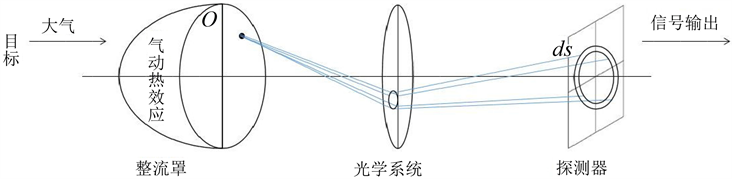Figure 1. Influence of aerodynamic thermal radiation from fairing on imaging

2.1. 整流罩的温度分布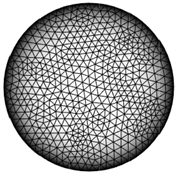Figure 2. Grid division of fairing model

2.1.1. 导热基本定理  

1) 导热过程：

$Q=\lambda \frac{\Delta t}{\delta }F$

Q为热量，单位为W；Δt为平壁两侧面的温度差，℃；δ为平壁的厚度，m；F为平壁的面积，m2；λ为导热系数。

2) 对流换热

$Q=\alpha \left({t}_{w}-{t}_{f}\right)$

${t}_{w},{t}_{f}$ 为壁面和流体的平均温度，单位为℃； $\partial$ 是平均对流换热系数。

3) 热流密度

$\left(-{q}_{w}\right)={\left(\frac{\mu }{{P}_{r}}\frac{\partial h}{\partial y}\right)}_{w}+{\left[\frac{\mu }{{P}_{r}}\left(Le-1\right)\underset{i}{\sum }{h}_{i}\frac{\partial {C}_{i}}{\partial y}\right]}_{w}$

${P}_{r}$ 称为普朗特数。

4) 传热过程 

$K=1/\left(\frac{1}{{h}_{1}}+\frac{\delta }{\lambda }+\frac{1}{{h}_{2}}\right)$

K——传热系数，W/(m2∙℃)； ${h}_{1},{h}_{2}$ ——围护结构两表面热交换系数，W/(m2∙℃)。

2.1.2. 仿真过程

$\begin{array}{c}{T}_{r,\theta ,\phi }^{k+1}=\frac{\alpha \Delta \tau }{\Delta {r}^{2}}\left({T}_{r-1,\theta ,\phi }^{k}+{T}_{r+1,\theta ,\phi }^{k}\right)+\frac{2\alpha \Delta \tau }{r\cdot \Delta r}{T}_{r+1,\theta ,\phi }^{k}\\ +\frac{\alpha \Delta \tau }{{r}^{2}{\mathrm{sin}}^{2}\phi \Delta {\theta }^{2}}\left({T}_{r,\theta -1,\phi }^{k}+{T}_{r,\theta +1,\phi }^{k}\right)\\ +\frac{\alpha \Delta \tau }{{r}^{2}\Delta {\phi }^{2}}\left({T}_{r,\theta ,\phi -1}^{k}+{T}_{r,\theta ,\phi +1}^{k}\right)+\frac{\alpha \mathrm{cos}\phi \Delta \tau }{{r}^{2}\mathrm{sin}\phi \Delta \phi }{T}_{r,\theta ,\phi +1}^{k}\\ +\left(1-\frac{2\alpha \Delta \tau }{\Delta {r}^{2}}-\frac{2\alpha \Delta \tau }{r\cdot \Delta r}-\frac{2\alpha \Delta \tau }{{r}^{2}{\mathrm{sin}}^{2}\phi \Delta {\theta }^{2}}-\frac{2\alpha \Delta \tau }{{r}^{2}\Delta {\phi }^{2}}-\frac{\alpha \mathrm{cos}\phi \Delta \tau }{{r}^{2}\mathrm{sin}\phi \Delta \phi }\right){T}_{r,\theta ,\phi }^{k}\end{array}$

$\begin{array}{c}{T}_{r,\theta ,\phi }^{k+1}=\frac{h\Delta \tau }{\rho c\Delta r}{T}_{1}-\frac{\lambda \Delta \tau }{\rho c\Delta {r}^{2}}{T}_{r-1,\theta ,\phi }^{k}\\ -\frac{\lambda \Delta \tau }{\rho c{r}^{2}\mathrm{sin}\phi \Delta \theta \Delta \phi }{T}_{r,\theta ,\phi +1}^{k}-\frac{\lambda \Delta \tau }{\rho c{r}^{2}\mathrm{sin}\phi \Delta \theta \Delta \phi }{T}_{r,\theta +1,\phi }^{k}\\ +\left(1-\frac{h\Delta \tau }{\rho c\Delta r}+\frac{\lambda \Delta \tau }{\rho c\Delta {r}^{2}}+\frac{\lambda \Delta \tau }{\rho c{r}^{2}\mathrm{sin}\phi \Delta \theta \Delta \phi }+\frac{\lambda \Delta \tau }{\rho c{r}^{2}\mathrm{sin}\phi \Delta \theta \Delta \phi }\right){T}_{r,\theta ,\phi }^{k}\end{array}$

2.1.3. 仿真实验结果

2.2. 整流罩红外辐射计算  

2.2.1. 红外辐射理论基础

1) 普朗克定律

${M}_{b\lambda }=\frac{{c}_{1}}{{\lambda }^{5}}\frac{1}{{e}^{\frac{{c}_{2}}{\lambda T}}-1}$

2) 斯蒂芬–玻尔兹曼定律

$M={\int }_{0}^{\infty }{M}_{\lambda }\text{d}\lambda =\sigma {T}^{4}$

3) 距离平方反比定律

$E=\frac{\text{d}\Phi }{\text{d}A}=\frac{I}{{d}^{2}}$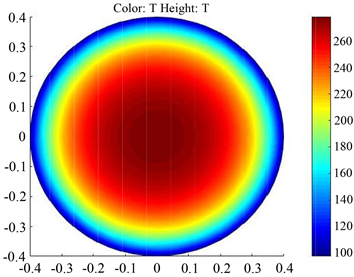(a) 1 Ma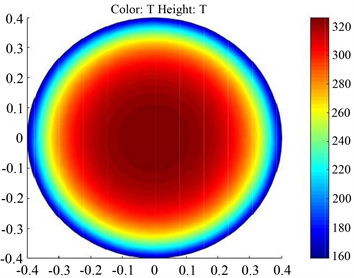(b) 1.5 Ma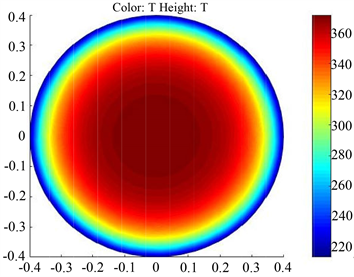(c) 2 Ma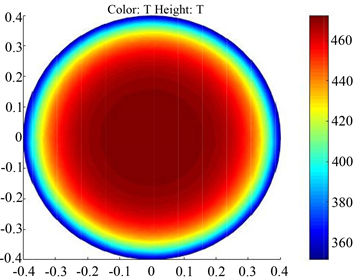(d) 2.5 Ma

Figure 3. Cloud diagram of fairing temperature at different speeds

4) 扩展源产生的辐射照度

$E=M$

2.2.2. 仿真过程

${\theta }_{1}=\mathrm{arctan}\left(\frac{R\mathrm{sin}\theta }{{d}_{1}+{d}_{2}-\left(R-R\mathrm{cos}\theta \right)}\right)$

$E=\frac{I\mathrm{cos}{\theta }_{2}\mathrm{cos}{\theta }_{1}}{{\left(\frac{R\mathrm{sin}\theta }{\mathrm{sin}{\theta }_{1}}\right)}^{2}}$

2.2.3. 仿真实验结果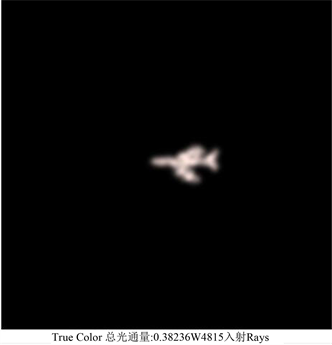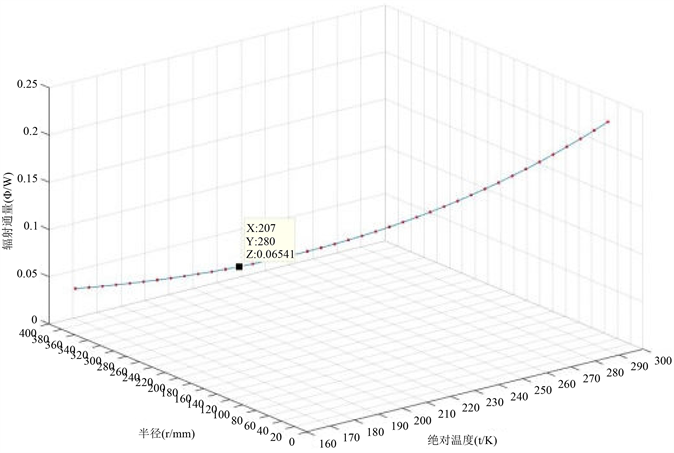(a) 288 K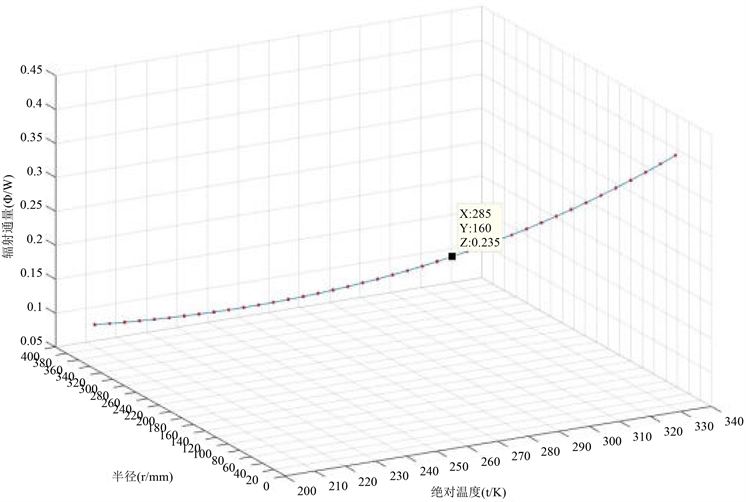(b) 330 K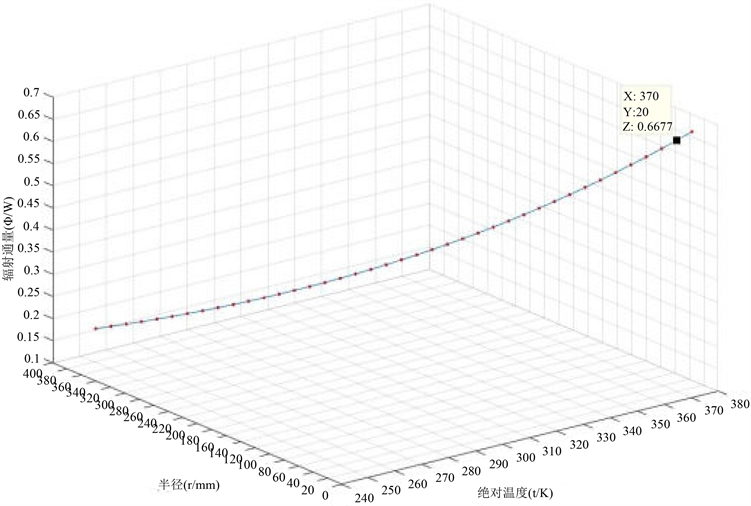(c) 373 K(d) 473 K

Figure 5. Coordinates of radiation intensity of the fairing at different temperatures

3. 整流罩红外辐射的建立与仿真

TracePro是一款基于蒙特卡罗法的非序列光线追迹软件，普遍用于照明系统、光学分析、辐射分析及光度分析。TracePro可采用“普适光线追迹”技术来追击辐射，可以引入一个辐射源辐射到一个设定好的模型上，在模型内部和界面处没有额外的损耗，在每个界面上，辐射遵从吸收、反射等定律。相对于传统的MATLAB软件建模仿真方式，TracePro软件可以仿真辐射在模型中不同的传播路径时，还可追踪其辐射通量以及辐射吸收量情况，特别是它能够对真实场景计算和显示。可以利用TracePro建立实体模型，设置辐射源、光学系统、探测器以及相关光学参数和辐射参数，对整个系统进行模拟仿真。辐射源是一切辐射系统的根本，所以首先就是建立辐射源，其次建立卡塞格林光学系统  ，最后设置一个接收面。本文将飞行器整流罩作为辐射源进行设计。

3.1. 整流罩红外辐射建立模型

3.1.1. 辐射源建立

3.1.2. 光学系统建立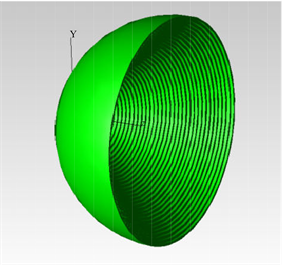Figure 6. Division of fairing radiation intensity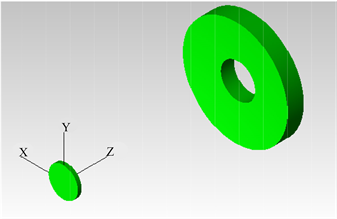Figure 7. Cassegrain optical system model

$\partial =\frac{{D}_{2}}{{D}_{1}}$

${{f}^{\prime }}_{1}=\frac{{r}_{o1}}{2}={f}_{1}$

${{f}^{\prime }}_{2}=\frac{{r}_{o2}}{2}={f}_{2}$

${f}^{\prime }=-\frac{{{f}^{\prime }}_{1}{{f}^{\prime }}_{2}}{\Delta }=\frac{{{f}^{\prime }}_{1}{{f}^{\prime }}_{2}}{{{f}^{\prime }}_{1}-{{f}^{\prime }}_{2}-d}=f$

3.2. 整流罩红外辐射仿真过程

3.3. 仿真结果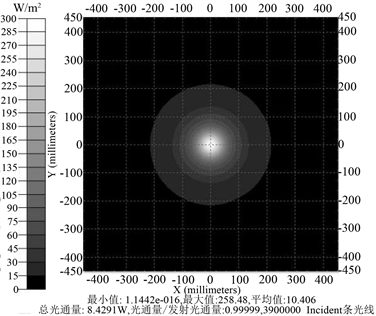(a) 288 K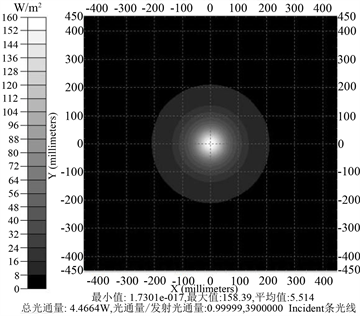(b) 330 K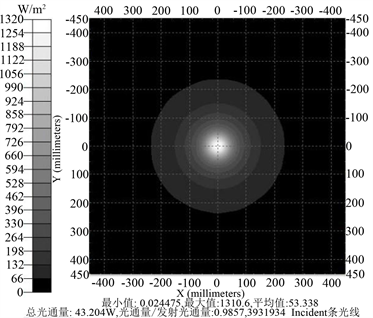(c) 373 K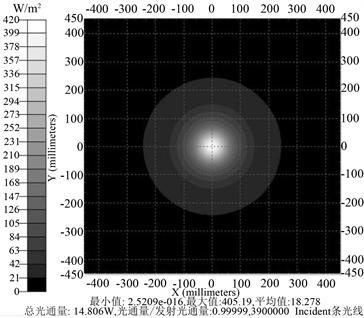(d) 473 K

Figure 8. Analysis diagram of fairing radiation intensity at different temperatures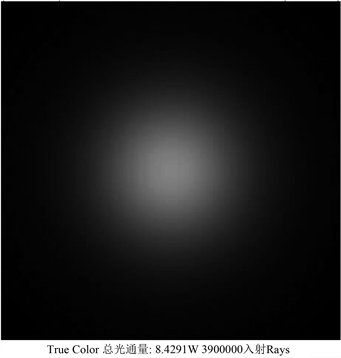(a) 288 K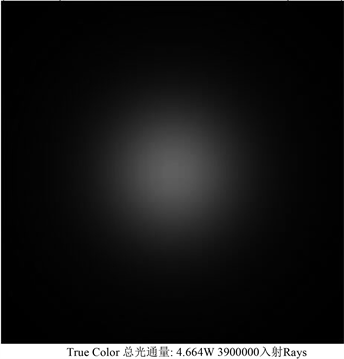(b) 330 K(c) 373 K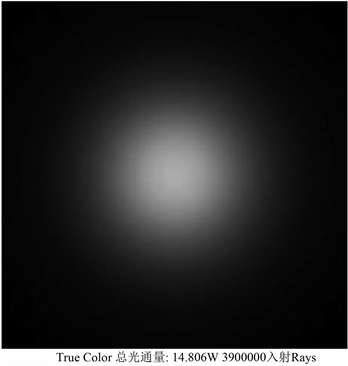(d) 473 K

Figure 9. Radiation intensity of the fairing at different temperatures

4. 整流罩热噪声对成像的影响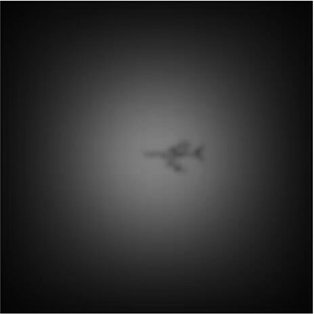(a) 288 K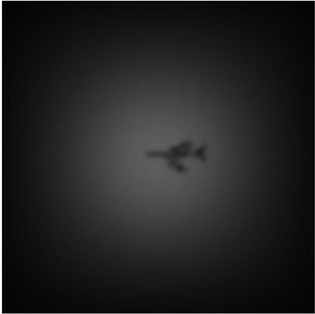(b) 330 K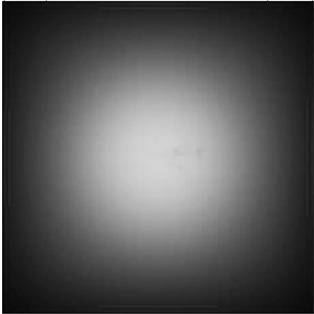(c) 373 K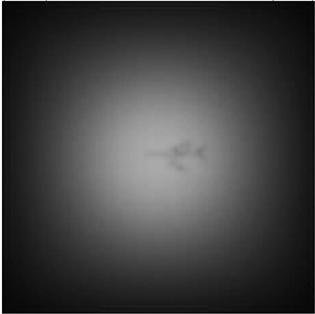(d) 473 K

Figure 10. Target infrared simulation images at different fairing temperatures

5. 总结

Imaging Simulation under the Background of Strong Infrared Thermal Radiation[J]. 建模与仿真, 2019, 08(02): 60-72. https://doi.org/10.12677/MOS.2019.82009

1. 1. 高希磊. 整流罩气动加热对成像影响建模与仿真[D]: [硕士学位论文]. 西安: 西安电子科技大学, 2013.

2. 2. 罗大雷. 高超、超声速飞行器头部激波层红外辐射特性数值研究[D]: [硕士学位论文]. 长沙: 国防科学技术大学研究生院, 2012.

3. 3. 葛永博. 空中目标红外辐射建模及成像仿真[D]: [硕士学位论文]. 哈尔滨: 哈尔滨工业大学, 2015.

4. 4. 田杰. 目标红外辐射特征与建模仿真技术研究[D]: [硕士学位论文]. 南京: 南京理工大学, 2016.

5. 5. 薛文慧. 气动热环境下共形整流罩热辐射特性研究[D]: [硕士学位论文]. 哈尔滨: 哈尔滨工业大学, 2016.

6. 6. 王霄. 空间目标红外成像建模与仿真[D]: [硕士学位论文]. 北京: 中国科学院大学, 2017.

7. 7. 许求真. 经典卡塞格林系统热差分析[J]. 激光与红外, 2011, 41(4): 436-441.

8. 8. 孙海锋. 高速飞机红外成像过程的数值模拟与特性分析[D]: [博士学位论文]. 哈尔滨: 哈尔滨工业大学, 2018.

9. 9. 李振. 预警机蒙皮红外特征与成像仿真技术研究[D]: [硕士学位论文]. 成都: 电子科技大学, 2016.

10. NOTES

*通讯作者。